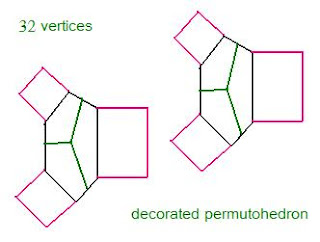Recall that the third theta function in the j invariant numerator, namely $\theta_{10}(0, \tau)$, transforms under $\tau \mapsto \tau + 1$ to $\theta_{10}(0, \tau)$ multiplied by the 8th root of unity $\textrm{exp}(i \frac{\pi}{4})$. For the j invariant, the 8th root disappears with the power of 8. Perhaps we should think of the power of 8 here as representing 8 vertices of a cube, where 3 cubes are formed from the six squares of a permutohedron, each paired to its opposite. Every vertex of such a cube is obtained by rotating the octahedron dual by $\textrm{exp}(i \frac{\pi}{4})$, as mentioned yesterday.
But why would one try to associate the j invariant with such low dimensional polytopes rather than, say, the $E8$ or Leech lattices? Why not! It is far easier to visualise what is going on in three dimensions, because that happens to be the dimension of our classical space template. And for 6 dimensional twistor type moduli, perhaps we can put 3 and 3 together.